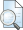## FLOW ANALYSIS ON PIPE DISTRIBUTION NETWORK USING DIFFERENTIAL EVOLUTION ALGORITHM (DE)

Sulianto, Sulianto (2015) FLOW ANALYSIS ON PIPE DISTRIBUTION NETWORK USING DIFFERENTIAL EVOLUTION ALGORITHM (DE). In: Proceeding of The 1st Young Scientist International Conference of Water Resources Development and Environmental Protection 2015 (ICWRDEP 2015). Jurusan Teknik Pengairan Fakultas Teknik Universitas Brawijaya, B54-B62.Preview
Text
Sulianto - differential evolution network pipe.pdfPreview
Text
Peer Review - Sulianto - differential evolution network pipe.pdfPreview
Text
Similarity - Sulianto - differential evolution network pipe.pdf

## Abstract

The hydraulic of equations system in the water distribution network is a non-linear equations system so as to complete it requires iteration technique. The method is often applied to solve this problem is the Hardy-Cross Method and Newton Raphson based on numerical methods. As the development of reliable enough metaheuristic method to solve optimization problems, this method also has the opportunity to be able to be applied to the completion of a hydraulic equations network system. Genetic Algorithm is a metaheuristic method most widely explored. This method can work well, but are also considered inefficient because it requires a relatively long time iteration. This paper tries to apply other metaheuristic method, namely Differential Evolution (DE). In the application of this method, the energy absolute at each node into the optimum variable to be searched, with the objective function of minimizing the deviation from the value of the discharge demand analysis results and discharge targeted at the needs of each service node. From the analysis of network pipe that has 12 elements and 10 nodes service, DE may indicate a fairly good performance. With the input parameters of the individual in a population of 800 and a maximum of 500 iterations of the obtained value of the root mean square error of 0.04 liters/second, equivalent to an average error rate of 5.90%. When compared with the results obtained from the Newton Raphson method, the mean energy absolute deviation of 4.96%, loss of energy at element 8.11 % and vector flow in the pipe at 4.34%.

Item Type: Book Section / Proceedings differential evolution, networks, pipe T Technology > TA Engineering (General). Civil engineering (General) Faculty of Engineering > Department of Civil Engineering (22201) Dr. Ir. MT Sulianto Sulianto 21 Sep 2020 09:17 22 Jun 2021 07:17 http://eprints.umm.ac.id/id/eprint/66680View Item